signed

QiShunwang

“诚信为本、客户至上”

# cs224w（图机器学习）2021冬季课程学习笔记4 Link Analysis: PageRank (Graph as Matrix)

2021/6/3 13:52:33   来源：

cs224w（图机器学习）2021冬季课程学习笔记集合

### 文章目录

• 1. Graph as Matrix
• 2. PageRank / the Google Algorithm
• 2.1 PageRank: The "Flow" Model
• 2.2 PageRank: Matrix Formulation
• 2.3 Connection to Random Walk
• 2.4 Eigenvector Formulation
• 3. PageRank: How to solve?
• 4. Random Walk with Restarts & Personalized PageRank
• 5. Matrix Factorization and Node Embeddings
• 6. 本章总结
• 7. 文中及脚注未提及的其他参考资料

PageRank是一种衡量网络中节点重要性的指标，主要思想是如果一个节点被很多重要节点指向，那么该节点也很重要。

M / G 是随机游走的概率转移矩阵。

Personalized PageRank和Random Walk with Restarts（可用于衡量图中节点间相似性，如应用于推荐问题）：主要区别在于teleport sets。

# 1. Graph as Matrix

1. 本节课研究矩阵角度的图分析和学习。
2. 这里的矩阵就是指邻接矩阵。
3. 将图视为矩阵形式，可以通过随机游走的方式定义节点重要性（即PageRank），通过矩阵分解matrix factorization (MF)来获取节点嵌入，将其他节点嵌入（如node2vec）也视作MF。

# 2. PageRank / the Google Algorithm

1. PageRank是谷歌搜索用的算法，用于对网页的重要性进行排序。在搜索引擎应用中，可以对网页重要性进行排序，从而辅助搜索引擎结果的网页排名。
2. 在现实世界中，将整个互联网视作图：将网页视作节点，将网页间的超链接视作边有一些问题会影响我们如何定义节点（但是本节课暂时不考虑这些问题）：
1. Dynamic pages created on the fly2
2. dark matter：不可达（如有密码等）的database generated pages
3. 一个网页之间互相链接的情况的示例：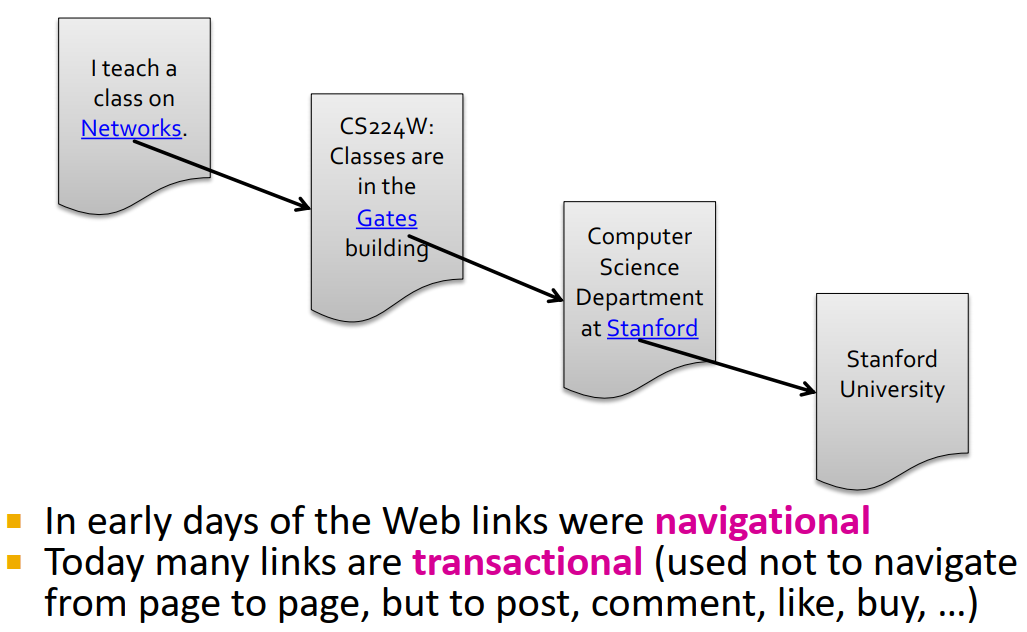4. 将网页看作有向图，以链接指向作为边的方向（这个网页/节点能直接跳转到的网页就作为其下一个节点successor）：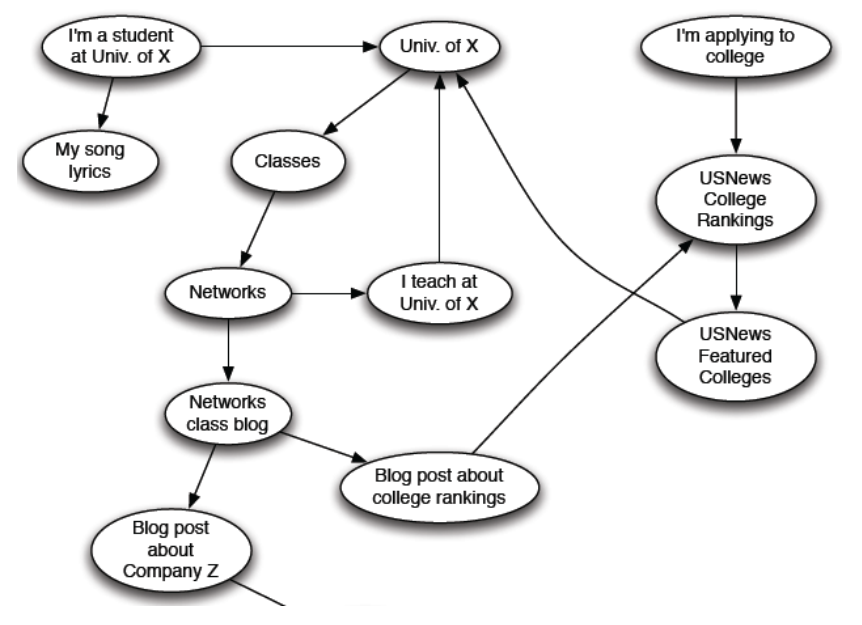5. 其他可表现为有向图形式的信息网络示例：论文引用，百科全书中词条间的互相引用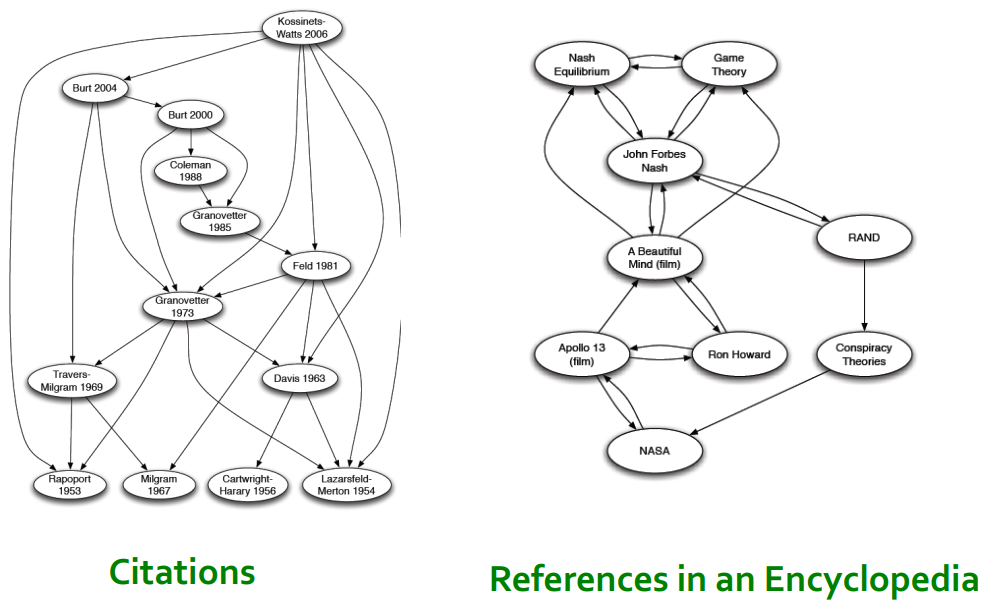6. 在图中，我们想要定义节点的重要性importance，通过网络图链接结构来为网页按重要性分级rank。以下将介绍3种用以计算图中节点重要性的方法：
1. PageRank
2. Personalized PageRank (PPR)
3. Random Walk with Restarts (RWR)
7. 衡量节点重要性：认为一个节点的链接越多，那么这个节点越重要。
这就成了一个递归recursive的问题——要计算一个节点的重要性就要先计算其predecessors的重要性，计算这些predecessors的重要性又要先计算它们predecessors的重要性……

## 2.1 PageRank: The “Flow” Model

1. 链接权重与其source page的重要性成正比例
2. 如果网页 i i 的重要性是 r i r_i ，有 d i d_i 个out-links，那么每个边的权重就是 r i / d i r_i/d_i
3. 网页 j j 的重要性 r j r_j 是其in-links上权重的总和4. 从而得到对节点 j j 的级别 r j r_j 的定义： r j = ∑ i → j r i d i r_j=\sum\limits_{i\rightarrow j}\frac{r_i}{d_i} d i d_i 是节点 i i 的出度）
5. 以这个1839年的网络为例：我们就可以得到这样的"flow" equations:
r y = r y / 2 + r a / 2 r_y=r_y/2+r_a/2
r a = r y / 2 + r m r_a=r_y/2+r_m
r m = r a / 2 r_m=r_a/2
6. 在直觉上我们好像可以用高斯消元法Gaussian elimination来解这个线性方程组，但这种方法不scalable。所以我们寻找更scalable的矩阵形式解法。

## 2.2 PageRank: Matrix Formulation

1. 建立随机邻接矩阵stochastic adjacency matrix M：网页 j j d j d_j 条out-links，如果 j → i j\rightarrow i M i j = 1 d j M_{ij}=\frac{1}{d_j}
M是列随机矩阵column stochastic matrix（列和为1）3
M的第 j j 列可以视作 j j 在邻居节点上的概率分布2. rank vector r r : 每个网页 i i 的重要性得分 r i r_i
∑ i r i = 1 \sum_i\mathbf{r}_i=1 （所以 r r 也可被视为是网络中所有节点的概率分布）
3. flow equations可以被写成： r = M ⋅ r \mathbf{r}=M\cdot \mathbf{r}
回忆一下原公式： r j = ∑ i → j r i d i r_j=\sum\limits_{i\rightarrow j}\frac{r_i}{d_i} （其实感觉从这个公式推到上个公式应该还是比较直觉的， M M 的第 j j 行被指向的节点对应的列的元素就是 1 / d i 1/d_i ，该列对应的是 r i \mathbf{r}_i ，加总起来就得到上个公式）
4. flow equation和矩阵对比的举例：## 2.3 Connection to Random Walk

1. 假想一个web surfer的随机游走过程，在 t 时间在网页 i i 上，在 t+1 时间从 i i 的out-links中随机选一条游走。如此重复过程。
向量 p ( t ) \mathbf{p}(t) 的第 i i 个坐标是 t 时间web surfer在网页 i i 的概率，因此向量 p ( t ) \mathbf{p}(t) 是网页间的概率分布向量。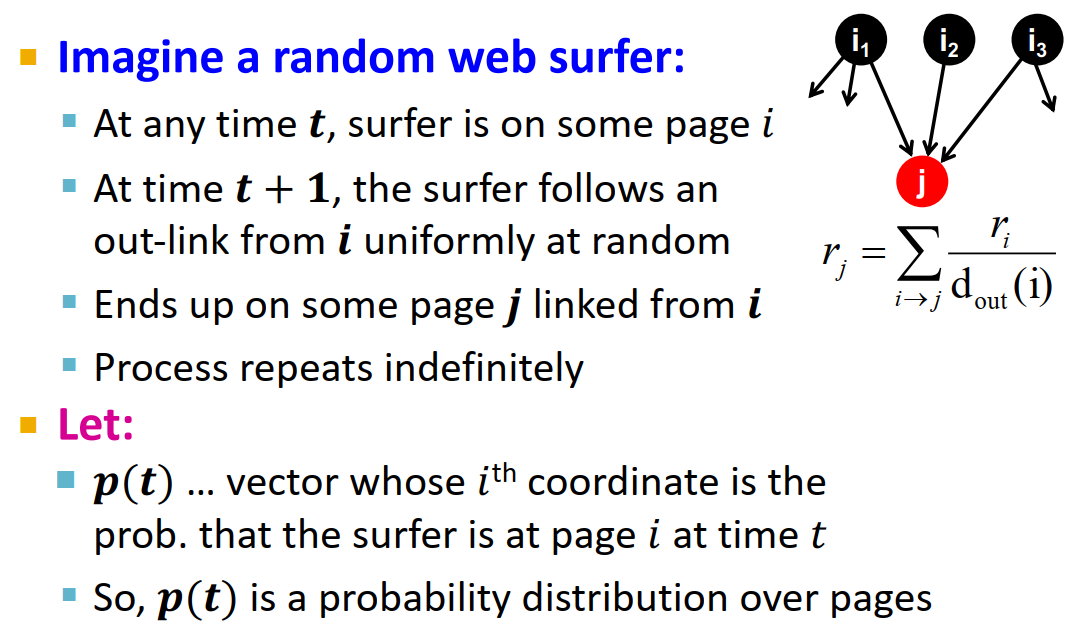2. 平稳分布stationary distribution
p ( t + 1 ) = M ⋅ p ( t ) \mathbf{p}(t+1)=M\cdot \mathbf{p}(t) （M是web surfer的转移概率，这个公式的逻辑感觉和 r = M ⋅ r \mathbf{r}=M\cdot \mathbf{r} 其实类似）
如果达到这种条件，即下一时刻的概率分布向量与上一时刻的相同： p ( t + 1 ) = M ⋅ p ( t ) = p ( t ) \mathbf{p}(t+1)=M\cdot \mathbf{p}(t)=\mathbf{p}(t) p ( t ) \mathbf{p}(t) 是随机游走的stationary distribution
r = M ⋅ r \mathbf{r}=M\cdot \mathbf{r} ，所以 r \mathbf{r} 是随机游走的stationary distribution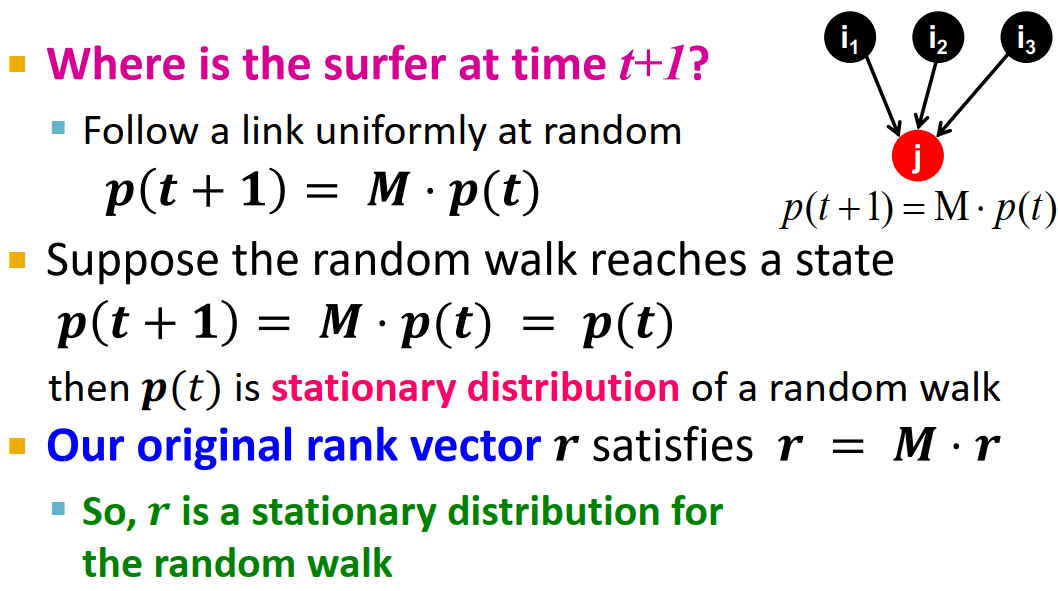这种做法将PageRank与随机游走概念进行了联合

## 2.4 Eigenvector Formulation

1. 无向图的邻接矩阵的特征向量是节点特征eigenvector centrality4，而PageRank定义在有向图的随机邻接矩阵上。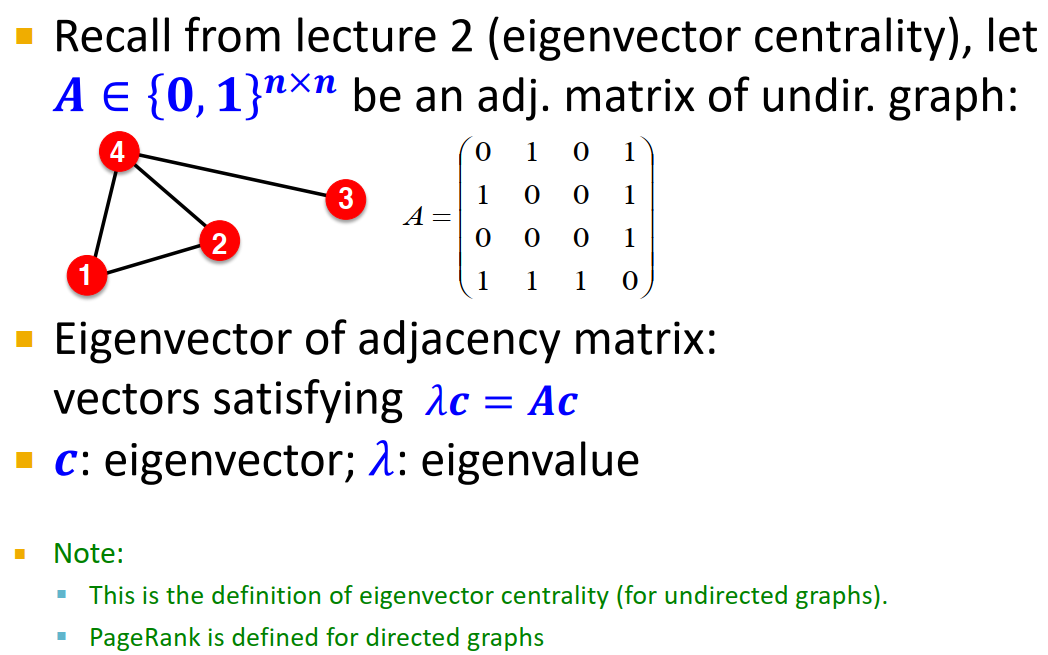2. 1 ⋅ r = M ⋅ r 1\cdot\mathbf{r}=M\cdot\mathbf{r}
rank vector r \mathbf{r} 是随机邻接矩阵M的特征向量，对应特征值为1。
从任一向量 u \mathbf{u} 开始，极限 M ( M ( . . . M ( M u ) ) ) M(M(...M(M\mathbf{u}))) 是web surfer的长期分布，PageRank=极限分布=M的principal eigenvector5
根据这个思路，我们就能找到PageRank的求解方式：power iteration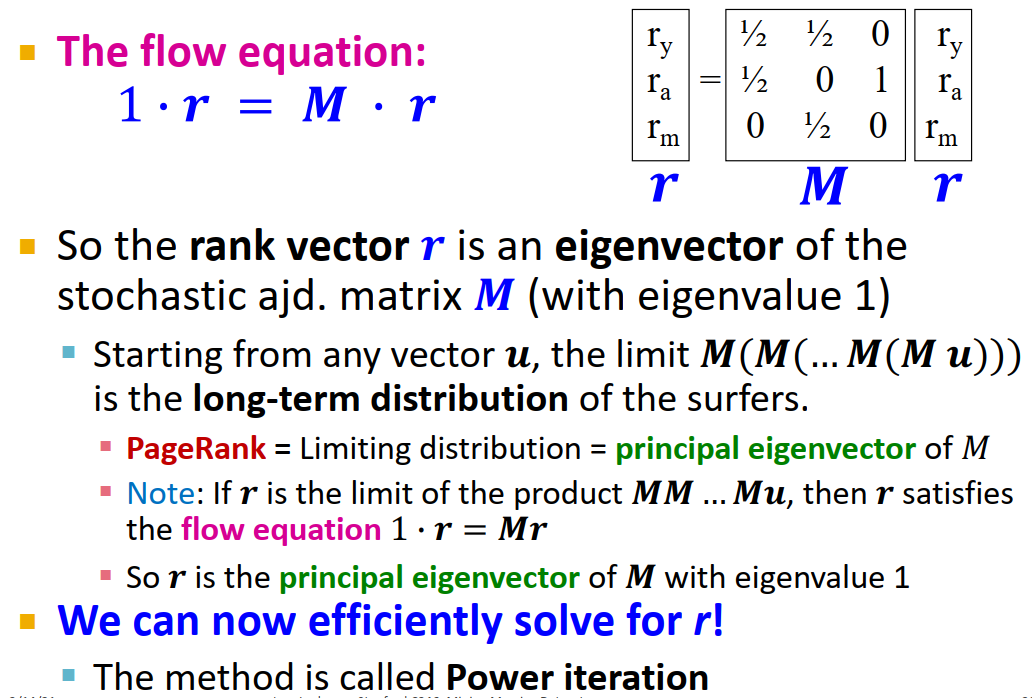limit极限
limiting distribution极限分布
6
相当于是random surfer一直随机游走，直至收敛，到达稳定状态。
这个M的叠乘可以让人联想到Katz index叠乘邻接矩阵A7
相比高斯消元法，power iteration是一种scalable的求解PageRank方法。
3. PageRank总结
1. 通过网络链接结构衡量图中节点的重要性
2. 用随机邻接矩阵M建立web surfer随机游走模型
3. PageRank解方程： r = M ⋅ r \mathbf{r}=M\cdot \mathbf{r}
r \mathbf{r} 可被视作M的principle eigenvector，也可被视作图中随机游走的stationary distribution